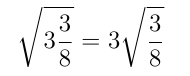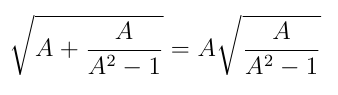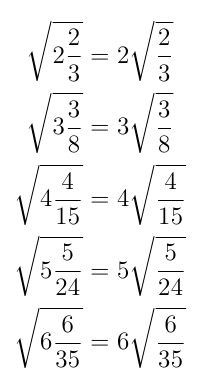# Beautiful Roots

This rather elegant fact has been going round on Twitter:

r
However, there are other examples of this phenomenon…

r
i.e.In fact, pleasingly, we can construct an infinite number of facts like this.

Let’s say that we want to find positive integers A, B and C, such that:

√(A + B/C) = A √(B/C)                                                               

We can also suppose that B < C (otherwise our mixed fraction would be a little odd) and that the highest common factor of B and C is 1, so that the fraction B/C is in its lowest form.

To find a relationship between A, B and C, we can square  and rearrange the equation to get the A terms on one side:

___________________________   ⇔           A + B/C = A² (B/C)
_______________________________⇔                      A = (A² – 1) (B/C)
_______________________________⇔         A/(A² – 1) = B/C    ,   A > 1                                                        

We can use double implications (⇔) here since we know that everything is positive.

Now,  tells us that two fractions with positive integer numerator and denominator are equal. Both fractions are in their lowest form (B/C because we said so earlier, while A and (A²1) clearly have a highest common factor of 1). Therefore the numerators and denominators are equal:

   ⇔   A = B   and  A² – 1 = C   (if A > 1)

This means that there is an infinite family of solutions of the form:Any integer greater than 1 will do as a value for A, so we have:and so on…

Rather nice.

Click HERE for another investigation of a maths tweet from Cliff Pickover.

## 3 thoughts on “Beautiful Roots”

1.Perry Leaves

In these examples there should be a “+” symbol between the integer and the fraction that appear under the radical. Some one should correct this!

1.thomas Post author

It is implied summation on the left hand side, following the (somewhat idiosyncratic) convention of how mixed numbers are presented, but implied multiplication on the right hand side, following the convention of everything else in mathematics. I think it is correct as stated.

2. Pingback: Mixed Number Curiosity | CAS Musings